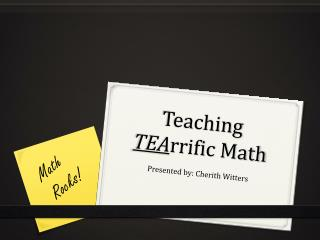Download PresentationTeaching TEA rrific Math

# Teaching TEA rrific Math

Download Presentation## Teaching TEA rrific Math

- - - - - - - - - - - - - - - - - - - - - - - - - - - E N D - - - - - - - - - - - - - - - - - - - - - - - - - - -
##### Presentation Transcript

1. Teaching TEArrific Math Math Rocks! Presented by: CherithWitters

2. T Take the fear out of math! * Positive Talk * Provide Support – Math Notebook * Saving students one brain at a time!

3. E Energetic Teaching! * Go ahead…make that mistake * Get off the stage * PPT – the secret weapon * Choose wisely

4. A Active Engagement! * Build your own games * Jeopardy * Group Challenges * Gadgets and Gizmos

5. Common Core VS Michigan Outcomes http://www.corestandards.org/ comparing michigan math standards with common core htthttp://www.michigan.gov/documents/mde/K-8_GLCE_Mathematics_-_CCS_Alignment_sc_4-23-10_319495_7.pdfp://www.corestandards.org/

6. Number—Fractions 5-NF Operations on fractions 6. Understand that multiplying a fraction by a/b means taking a parts of a decomposition of the fraction into b equal parts. 7. Understand that the area of a rectangle with side lengths a/b and c/d is the product a/b × p/q. This extends the area formula for rectangles to fractional side lengths, and also allows products of fractions to be represented visually as areas of rectangles. 10. Calculate products of fractions, and quotients of unit fractions and nonzero whole numbers (with either as divisor), and solve word problems involving these operations. Represent these operations using equations, area models and length models. 9. Understand division of unit fractions by whole numbers and division of whole numbers by unit fractions: a. Dividing a unit fraction 1/b by a whole number a results in a smaller unit fraction 1/a × b. (Using the inverse relationship between multiplication and division: 1/3 ÷ 2 = 1/6 because 1/6 × 2 = 1/3.) b. Dividing a whole number a by a unit fraction 1/b results in a greater whole number a × b. (Using the inverse relationship between multiplication and division: 2 ÷ 1/3 = 6 because 6 × 1/3 = 2.) TAKE A LOOK Multiply and divide fractions N.ME.05.12 Find the product of two unit fractions with small denominators using area model. N.MR.05.13 Divide a fraction by a whole number and a whole number by a fraction, using simple unit fractions.